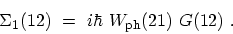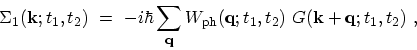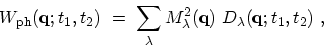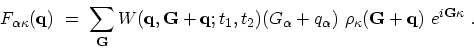## F.2.2 The Phonon Self-Energy

In the previous sections helpful forms of the electron-phonon interaction are derived. One can link up with the many-particle theory and introduce phonon contributions into the quantum kinetic equations. As shown in the derivation of the general result (F.37), one has to add the phonon induced contribution to the electronically screened interaction. Together with (F.14), this defines the phonon self-energies which enters the quantum kinetic equations. As the phonon induced interaction is not a functional of the single-particle GREEN's function, the problem is slightly less complicated than the electron-electron interaction. As in the purely electronic case, one is dependent on approximation schemes. An expansion of the self-energy in powers of the phonon-induced interaction is easily generated by means of (F.14). The contribution linear in the phonon induced interaction, i.e. the single-phonon self-energy takes the form(F.49)

By performing eigen-function expansion (see Appendix F.2.1) one obtains(F.50)

where(F.51)(F.52)(F.53)

The phonon GREEN's functions entering (F.51) are discussed in Appendix F.2.1.

M. Pourfath: Numerical Study of Quantum Transport in Carbon Nanotube-Based Transistors